# RD Sharma Solutions for Class 9 Maths Chapter 22 Tabular Representation of Statistical Data

## RD Sharma Solutions Class 9 Maths Chapter 22 – Free PDF Download

RD Sharma Solutions for Class 9 Maths Chapter 22 Tabular Representation of Statistical Data is given here which consists of questions and answers related to Statistics. This is the best study material for those students who are finding difficulties in solving problems. Our specialist teachers formulate these exercises to help you with your exam preparation to achieve good marks in Maths. RD Sharma Solutions are accurate and provide numerous methods of solving complex problems effortlessly. Students can download the PDF of this chapter for a better understanding of the concepts.

The solutions to all questions in RD Sharma books are given here in a detailed and step-by-step way to help the students understand more effectively. RD Sharma Solutions Class 9 is developed by professional teachers with the aim to enhance the interest in learning among students. Click on the link below to access all Class 9 Maths Chapter 22 – Tabular Representation of Statistical Data solutions free both online and offline.

## Download PDF of RD Sharma Solutions for Class 9 Maths Chapter 22 Tabular Representation of Statistical Data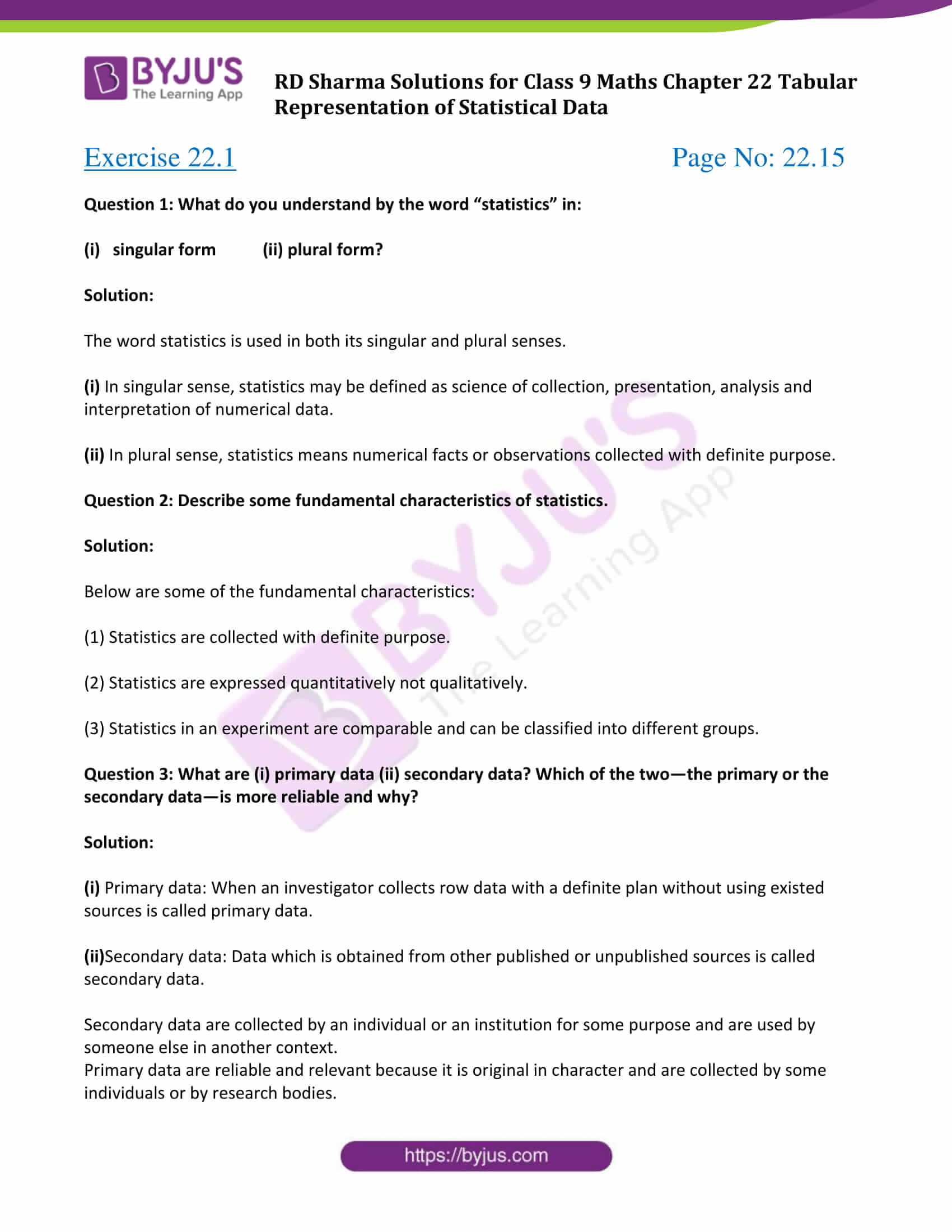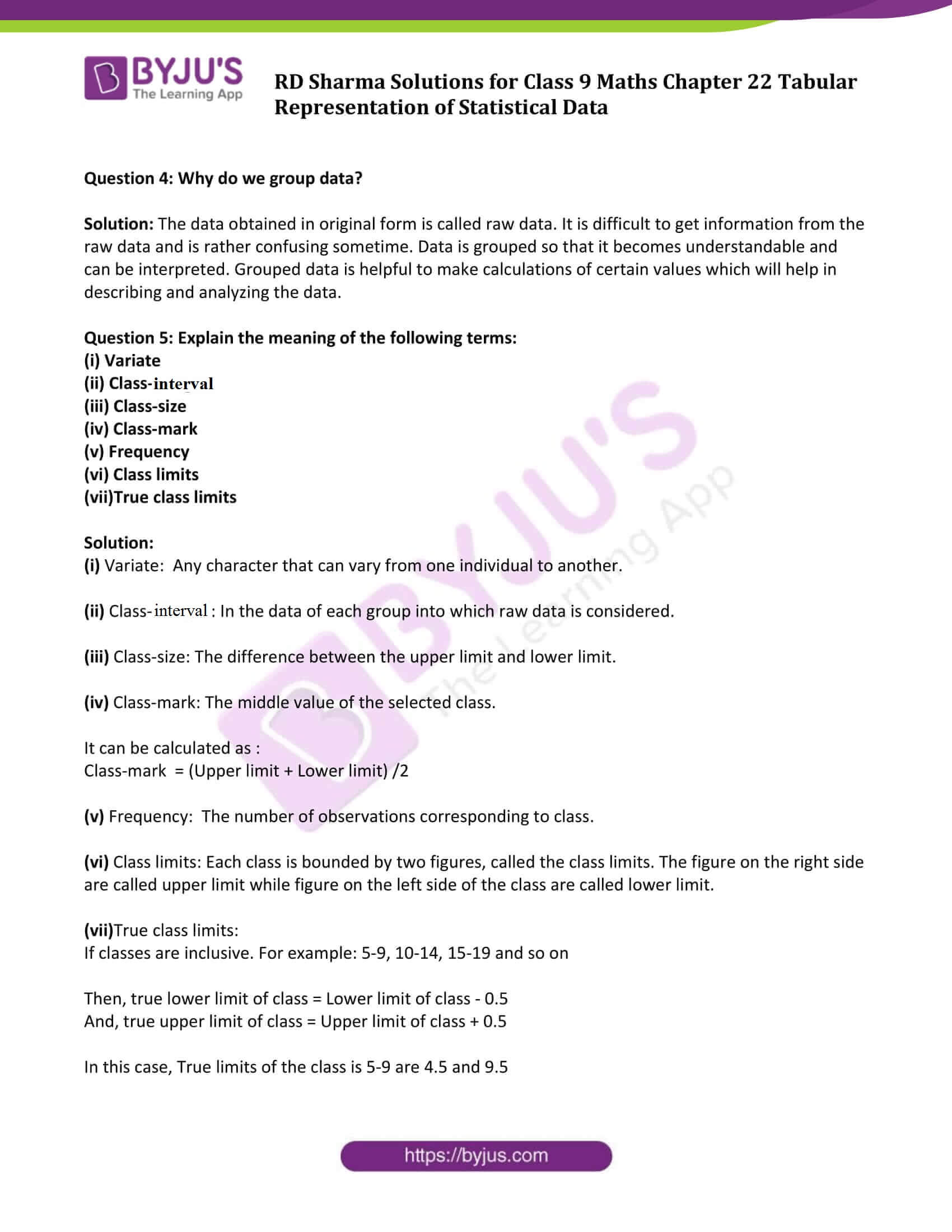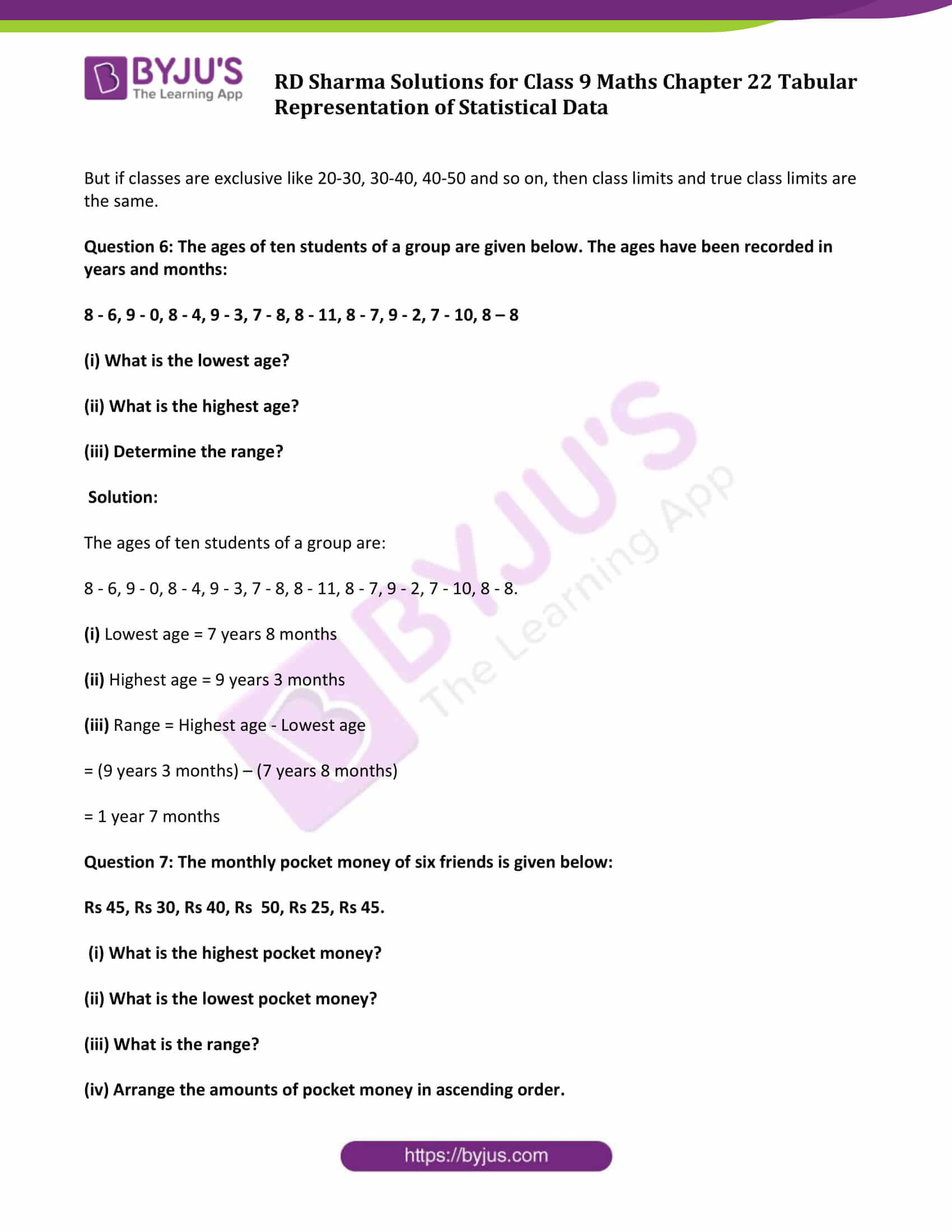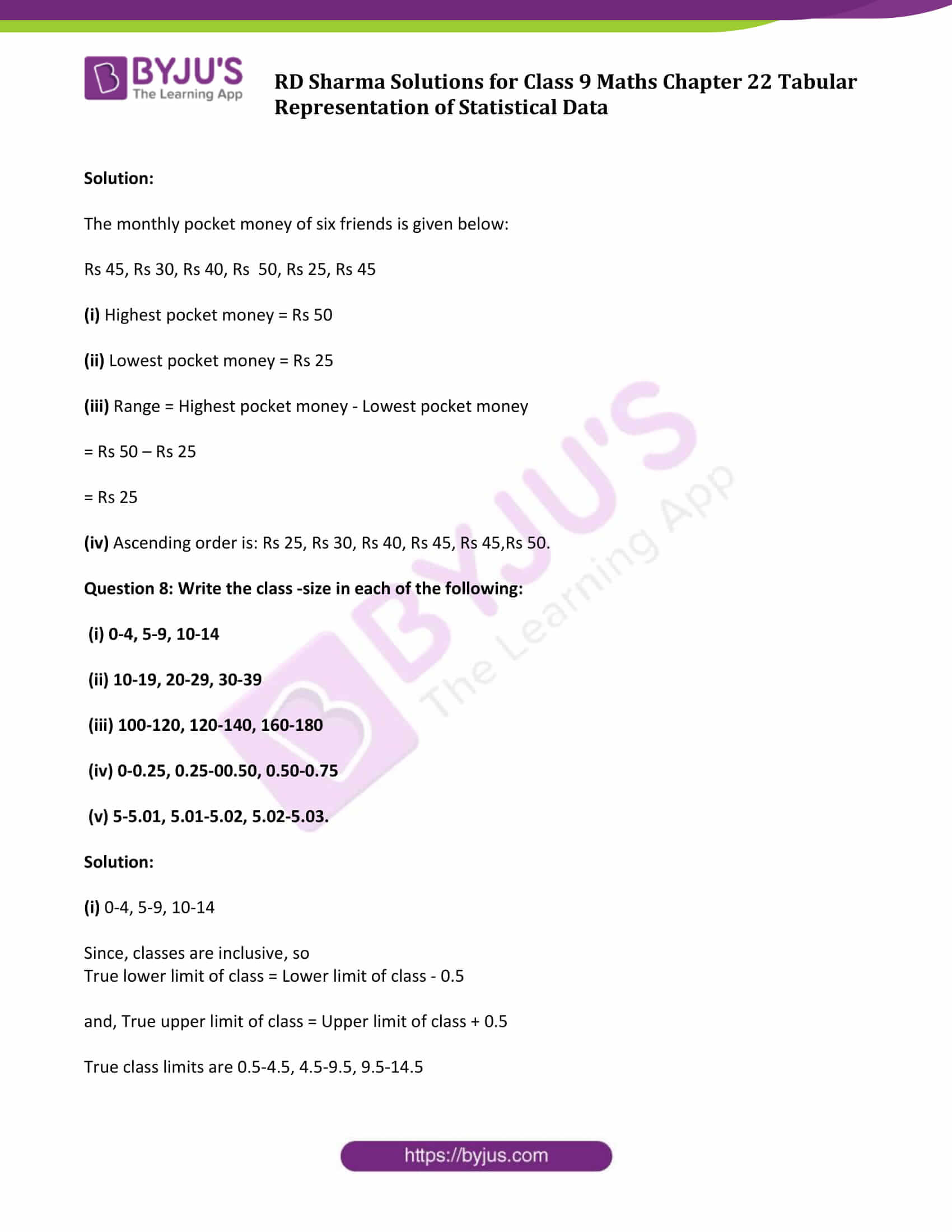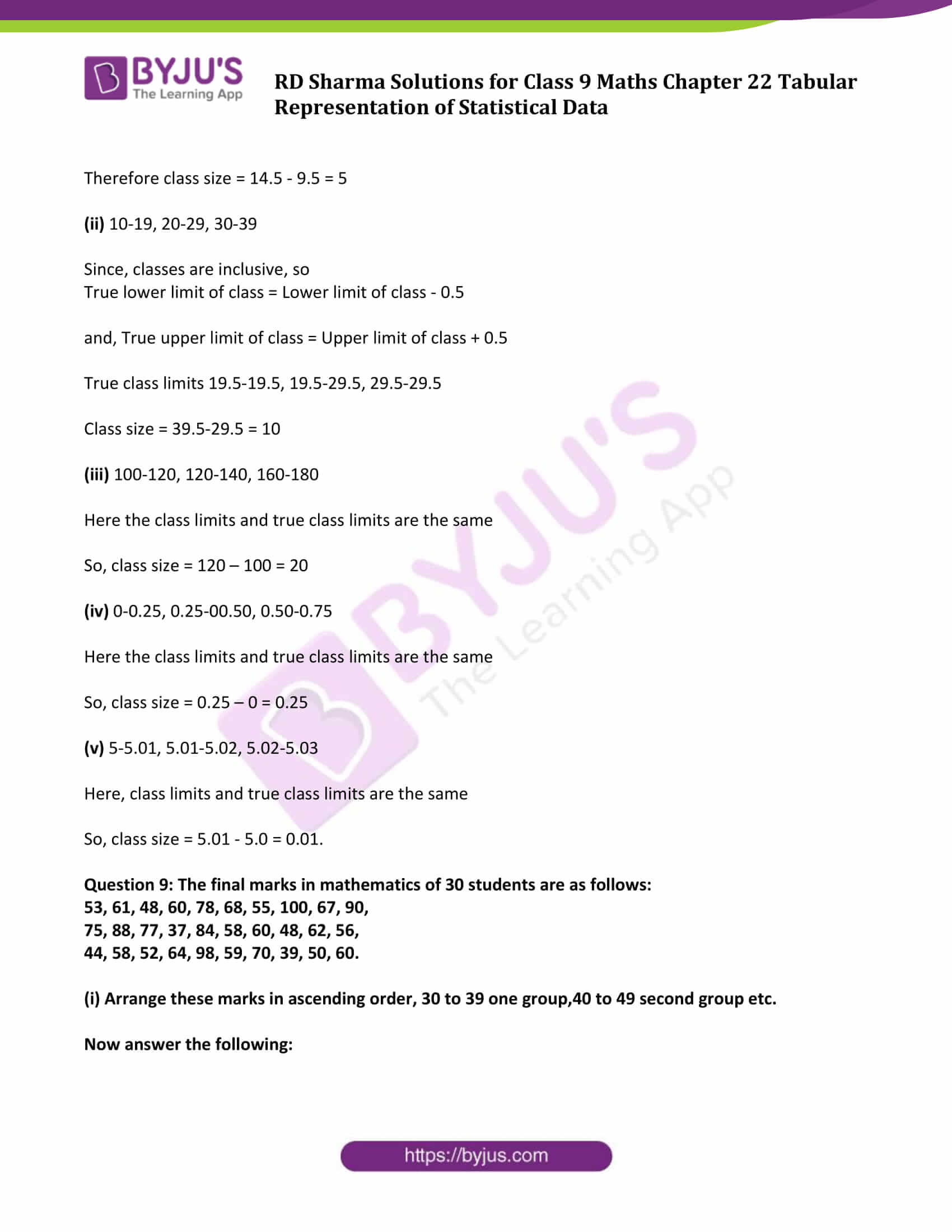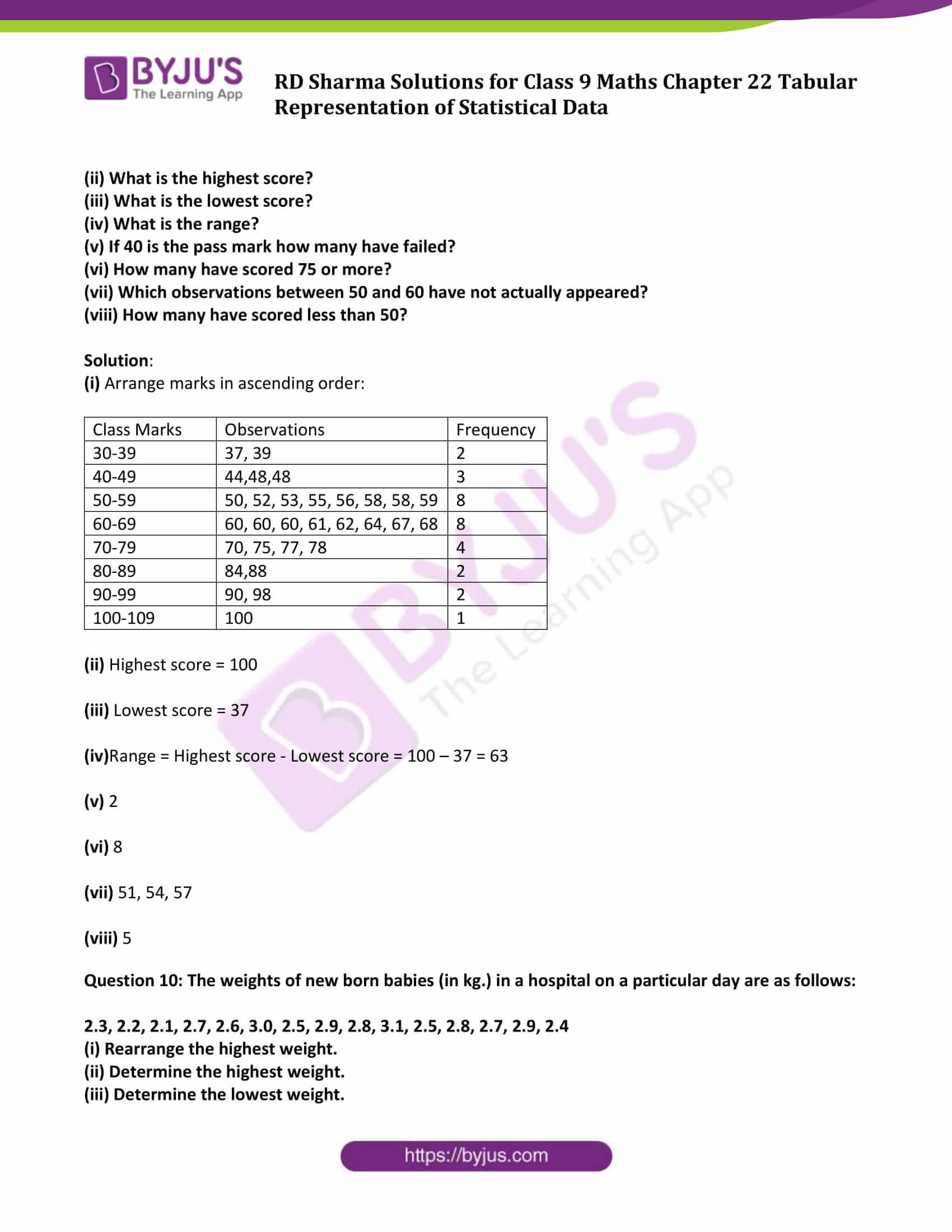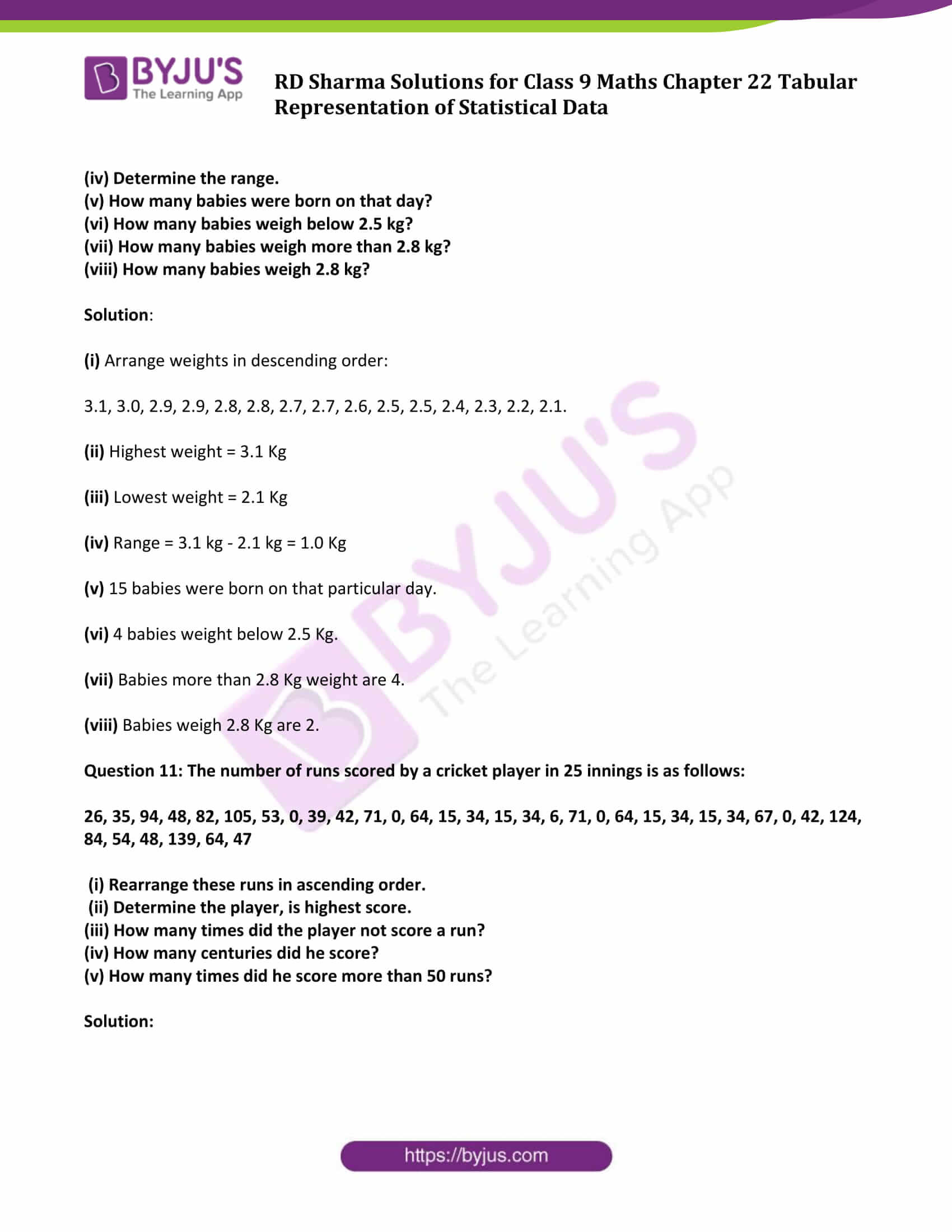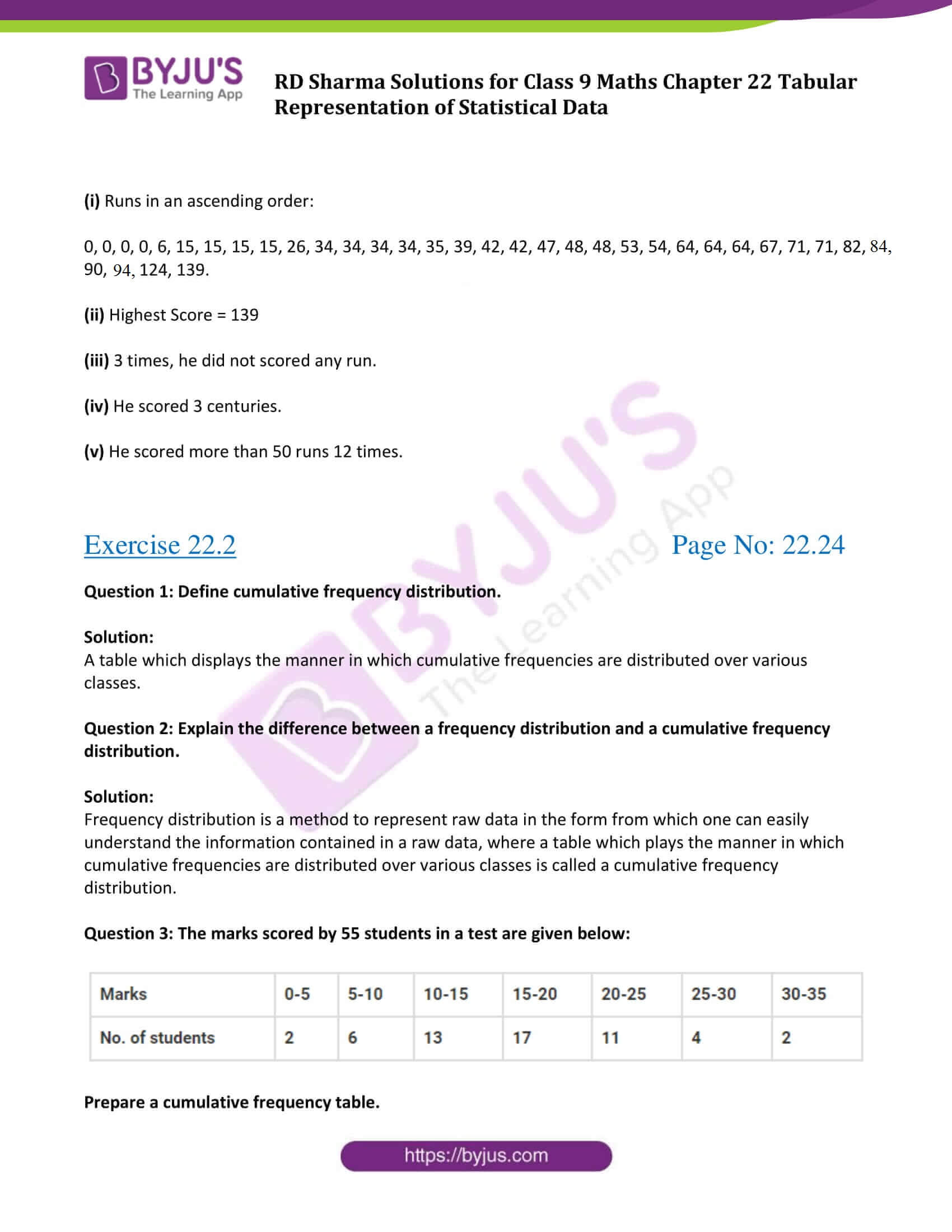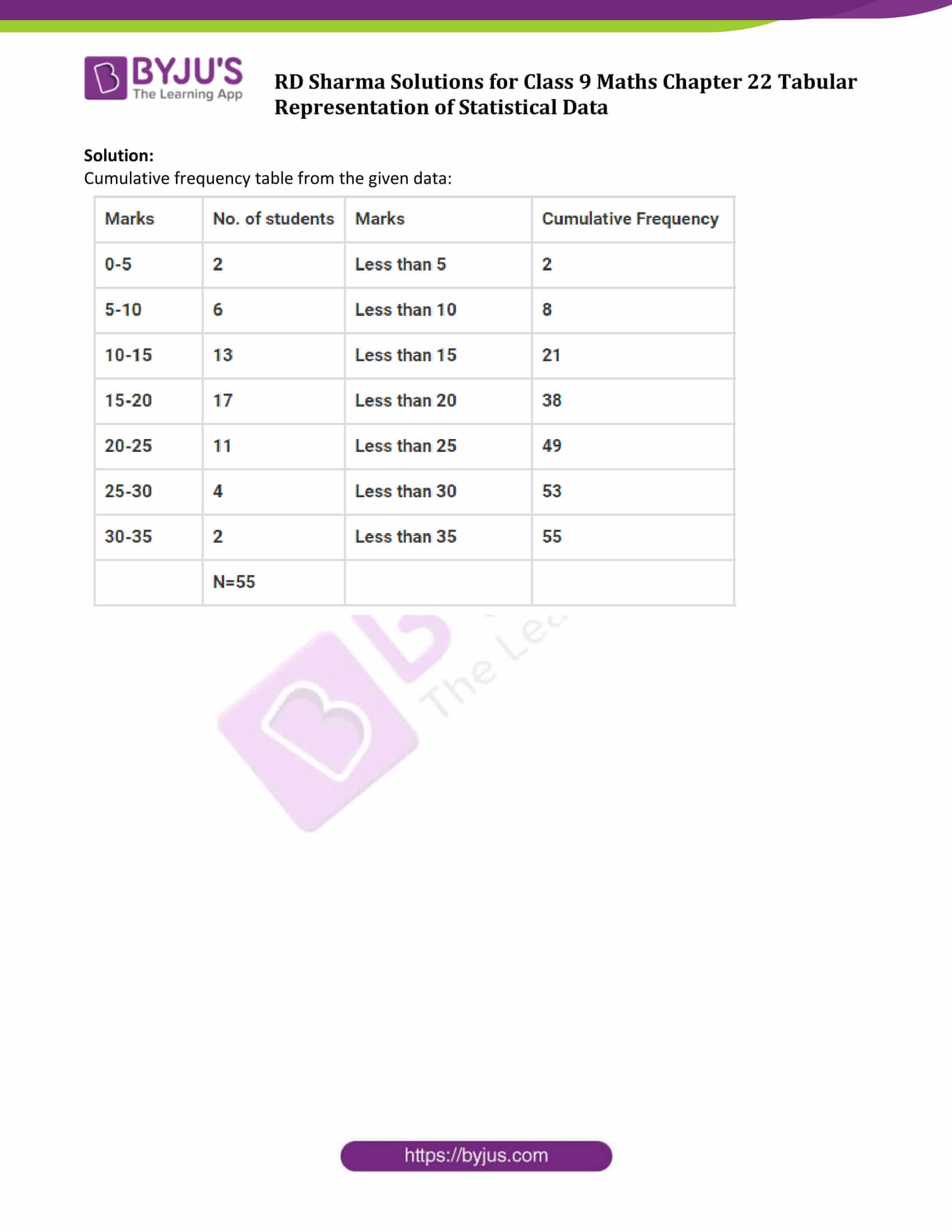### Exercise 22.1 Page No: 22.15

Question 1: What do you understand by the word “statistics” in:

(i) singular form (ii) plural form?

Solution:

The word statistics is used in both its singular and plural senses.

(i) In singular sense, statistics may be defined as science of collection, presentation, analysis and interpretation of numerical data.

(ii) In plural sense, statistics means numerical facts or observations collected with definite purpose.

Question 2: Describe some fundamental characteristics of statistics.

Solution:

Below are some of the fundamental characteristics:

(1) Statistics are collected with definite purpose.

(2) Statistics are expressed quantitatively not qualitatively.

(3) Statistics in an experiment are comparable and can be classified into different groups.

Question 3: What are (i) primary data (ii) secondary data? Which of the two—the primary or the secondary data—is more reliable and why?

Solution:

(i) Primary data: When an investigator collects row data with a definite plan without using existed sources is called primary data.

(ii)Secondary data: Data which is obtained from other published or unpublished sources is called secondary data.

Secondary data are collected by an individual or an institution for some purpose and are used by someone else in another context.

Primary data are reliable and relevant because it is original in character and are collected by some individuals or by research bodies.

Question 4: Why do we group data?

Solution: The data obtained in original form is called raw data. It is difficult to get information from the raw data and is rather confusing sometime. Data is grouped so that it becomes understandable and can be interpreted. Grouped data is helpful to make calculations of certain values which will help in describing and analyzing the data.

Question 5: Explain the meaning of the following terms:

(i) Variate

(ii) Class-interval

(iii) Class-size

(iv) Class-mark

(v) Frequency

(vi) Class limits

(vii)True class limits

Solution:

(i) Variate: Any character that can vary from one individual to another.

(ii) Class-interval: In the data of each group into which raw data is considered.

(iii) Class-size: The difference between the upper limit and lower limit.

(iv) Class-mark: The middle value of the selected class.

It can be calculated as :

Class-mark = (Upper limit + Lower limit) /2

(v) Frequency: The number of observations corresponding to class.

(vi) Class limits: Each class is bounded by two figures, called the class limits. The figure on the right side are called upper limit while figure on the left side of the class are called lower limit.

(vii)True class limits:

If classes are inclusive. For example: 5-9, 10-14, 15-19 and so on

Then, true lower limit of class = Lower limit of class – 0.5

And, true upper limit of class = Upper limit of class + 0.5

In this case, True limits of the class is 5-9 are 4.5 and 9.5

But if classes are exclusive like 20-30, 30-40, 40-50 and so on, then class limits and true class limits are the same.

Question 6: The ages of ten students of a group are given below. The ages have been recorded in years and months:

8 – 6, 9 – 0, 8 – 4, 9 – 3, 7 – 8, 8 – 11, 8 – 7, 9 – 2, 7 – 10, 8 – 8

(i) What is the lowest age?

(ii) What is the highest age?

(iii) Determine the range?

Solution:

The ages of ten students of a group are:

8 – 6, 9 – 0, 8 – 4, 9 – 3, 7 – 8, 8 – 11, 8 – 7, 9 – 2, 7 – 10, 8 – 8.

(i) Lowest age = 7 years 8 months

(ii) Highest age = 9 years 3 months

(iii) Range = Highest age – Lowest age

= (9 years 3 months) – (7 years 8 months)

= 1 year 7 months

Question 7: The monthly pocket money of six friends is given below:

Rs 45, Rs 30, Rs 40, Rs 50, Rs 25, Rs 45.

(i) What is the highest pocket money?

(ii) What is the lowest pocket money?

(iii) What is the range?

(iv) Arrange the amounts of pocket money in ascending order.

Solution:

The monthly pocket money of six friends is given below:

Rs 45, Rs 30, Rs 40, Rs 50, Rs 25, Rs 45

(i) Highest pocket money = Rs 50

(ii) Lowest pocket money = Rs 25

(iii) Range = Highest pocket money – Lowest pocket money

= Rs 50 – Rs 25

= Rs 25

(iv) Ascending order is: Rs 25, Rs 30, Rs 40, Rs 45, Rs 45,Rs 50.

Question 8: Write the class -size in each of the following:

(i) 0-4, 5-9, 10-14

(ii) 10-19, 20-29, 30-39

(iii) 100-120, 120-140, 160-180

(iv) 0-0.25, 0.25-00.50, 0.50-0.75

(v) 5-5.01, 5.01-5.02, 5.02-5.03.

Solution:

(i) 0-4, 5-9, 10-14

Since, classes are inclusive, so

True lower limit of class = Lower limit of class – 0.5

and, True upper limit of class = Upper limit of class + 0.5

True class limits are 0.5-4.5, 4.5-9.5, 9.5-14.5

Therefore class size = 14.5 – 9.5 = 5

(ii) 10-19, 20-29, 30-39

Since, classes are inclusive, so

True lower limit of class = Lower limit of class – 0.5

and, True upper limit of class = Upper limit of class + 0.5

True class limits 19.5-19.5, 19.5-29.5, 29.5-29.5

Class size = 39.5-29.5 = 10

(iii) 100-120, 120-140, 160-180

Here the class limits and true class limits are the same

So, class size = 120 – 100 = 20

(iv) 0-0.25, 0.25-00.50, 0.50-0.75

Here the class limits and true class limits are the same

So, class size = 0.25 – 0 = 0.25

(v) 5-5.01, 5.01-5.02, 5.02-5.03

Here, class limits and true class limits are the same

So, class size = 5.01 – 5.0 = 0.01.

Question 9: The final marks in mathematics of 30 students are as follows:

53, 61, 48, 60, 78, 68, 55, 100, 67, 90,

75, 88, 77, 37, 84, 58, 60, 48, 62, 56,

44, 58, 52, 64, 98, 59, 70, 39, 50, 60.

(i) Arrange these marks in ascending order, 30 to 39 one group,40 to 49 second group etc.

(ii) What is the highest score?

(iii) What is the lowest score?

(iv) What is the range?

(v) If 40 is the pass mark how many have failed?

(vi) How many have scored 75 or more?

(vii) Which observations between 50 and 60 have not actually appeared?

(viii) How many have scored less than 50?

Solution:

(i) Arrange marks in ascending order:

 Class Marks Observations Frequency 30-39 37, 39 2 40-49 44,48,48 3 50-59 50, 52, 53, 55, 56, 58, 58, 59 8 60-69 60, 60, 60, 61, 62, 64, 67, 68 8 70-79 70, 75, 77, 78 4 80-89 84,88 2 90-99 90, 98 2 100-109 100 1

(ii) Highest score = 100

(iii) Lowest score = 37

(iv)Range = Highest score – Lowest score = 100 – 37 = 63

(v) 2

(vi) 8

(vii) 51, 54, 57

(viii) 5

Question 10: The weights of new born babies (in kg.) in a hospital on a particular day are as follows:

2.3, 2.2, 2.1, 2.7, 2.6, 3.0, 2.5, 2.9, 2.8, 3.1, 2.5, 2.8, 2.7, 2.9, 2.4

(i) Rearrange the highest weight.

(ii) Determine the highest weight.

(iii) Determine the lowest weight.

(iv) Determine the range.

(v) How many babies were born on that day?

(vi) How many babies weigh below 2.5 kg?

(vii) How many babies weigh more than 2.8 kg?

(viii) How many babies weigh 2.8 kg?

Solution:

(i) Arrange weights in descending order:

3.1, 3.0, 2.9, 2.9, 2.8, 2.8, 2.7, 2.7, 2.6, 2.5, 2.5, 2.4, 2.3, 2.2, 2.1.

(ii) Highest weight = 3.1 Kg

(iii) Lowest weight = 2.1 Kg

(iv) Range = 3.1 kg – 2.1 kg = 1.0 Kg

(v) 15 babies were born on that particular day.

(vi) 4 babies weight below 2.5 Kg.

(vii) Babies more than 2.8 Kg weight are 4.

(viii) Babies weigh 2.8 Kg are 2.

Question 11: The number of runs scored by a cricket player in 25 innings is as follows:

26, 35, 94, 48, 82, 105, 53, 0, 39, 42, 71, 0, 64, 15, 34, 15, 34, 6, 71, 0, 64, 15, 34, 15, 34, 67, 0, 42, 124, 84, 54, 48, 139, 64, 47

(i) Rearrange these runs in ascending order.

(ii) Determine the player, is highest score.

(iii) How many times did the player not score a run?

(iv) How many centuries did he score?

(v) How many times did he score more than 50 runs?

Solution:

(i) Runs in an ascending order:

0, 0, 0, 0, 6, 15, 15, 15, 15, 26, 34, 34, 34, 34, 35, 39, 42, 42, 47, 48, 48, 53, 54, 64, 64, 64, 67, 71, 71, 82, 84, 90, 94, 124, 139.

(ii) Highest Score = 139

(iii) 3 times, he did not scored any run.

(iv) He scored 3 centuries.

(v) He scored more than 50 runs 12 times.

### Exercise 22.2 Page No: 22.24

Question 1: Define cumulative frequency distribution.

Solution:

A table which displays the manner in which cumulative frequencies are distributed over various classes.

Question 2: Explain the difference between a frequency distribution and a cumulative frequency distribution.

Solution:

Frequency distribution is a method to represent raw data in the form from which one can easily understand the information contained in a raw data, where a table which plays the manner in which cumulative frequencies are distributed over various classes is called a cumulative frequency distribution.

Question 3: The marks scored by 55 students in a test are given below: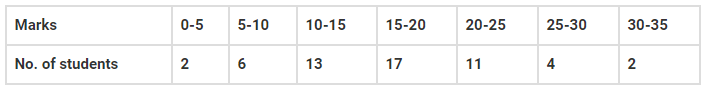Prepare a cumulative frequency table.

Solution:

Cumulative frequency table from the given data: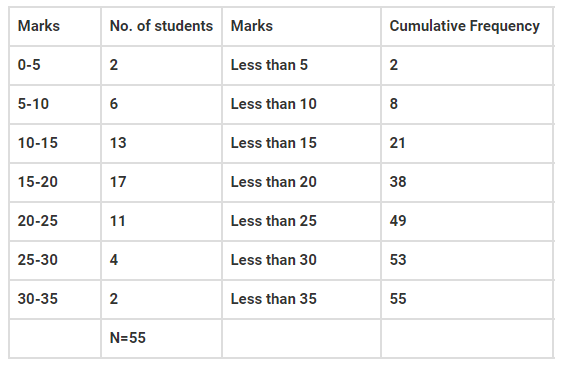### RD Sharma Solutions for Class 9 Maths Chapter 22 Tabular Representation of Statistical Data

In the 22nd Chapter of Class 9 RD Sharma Solutions students will study important concepts listed below:

• Statistics data
• Presentation of data
• Frequency distribution
• Construction of frequency distribution
• Cumulative frequency distribution

Statistics represent the sum of total observations. It is always expressed quantitatively not qualitatively. They are always collected with a definite purpose. In an experiment, statistics are comparable and can be classified into various groups.

Statistical data are of two types: Primary Data and Secondary Data

After the collection of data, the person has to find ways to arrange them in tabular form in order to observe their features and study them. Such an arrangement is called the presentation of data. The raw data can be arranged in any of the following ways:

(a) Ascending Order (b) Descending Order (c) Alphabetic Order

When the raw data is put in ascending or descending order of magnitude, it is called an array or arrayed data. This data can be studied using different methods of tally marks and frequency distribution. The number of times an observation occurs in the given data is called the frequency of the observation.

## Frequently Asked Questions on RD Sharma Solutions for Class 9 Maths Chapter 22

### Where can I get the accurate solutions for RD Sharma Solutions for Class 9 Maths Chapter 22?

Students can find the accurate answers in PDF format for Class 9 Maths RD Sharma Solutions at BYJU’S. Each and every question of the textbook has been designed accurately by Mathematics experts at BYJU’S. All these solutions are provided by considering the new pattern of the CBSE syllabus so that students can get thorough knowledge for their exams and secure good marks.

### What is the meaning of statistics according to RD Sharma Solutions for Class 9 Maths Chapter 22?

According to RD Sharma Solutions for Class 9 Maths Chapter 22 Statistics represent a sum of total observations. It is always expressed quantitatively not qualitatively. They are always collected with a definite purpose. In an experiment, statistics are comparable and can be classified into various groups.

### Is it necessary to learn all the questions provided in RD Sharma Solutions for Class 9 Maths Chapter 22?

Yes, as all types of questions are important from an exam perspective you must learn all of them. By learning all the solutions you can score high in Maths board exams and also grasp concepts flawlessly. Diligent practice of these solutions provides thorough knowledge of each concept and boosts confidence to appear for board exams fearlessly.